# Answers Chapter Solutions Test Paper – 1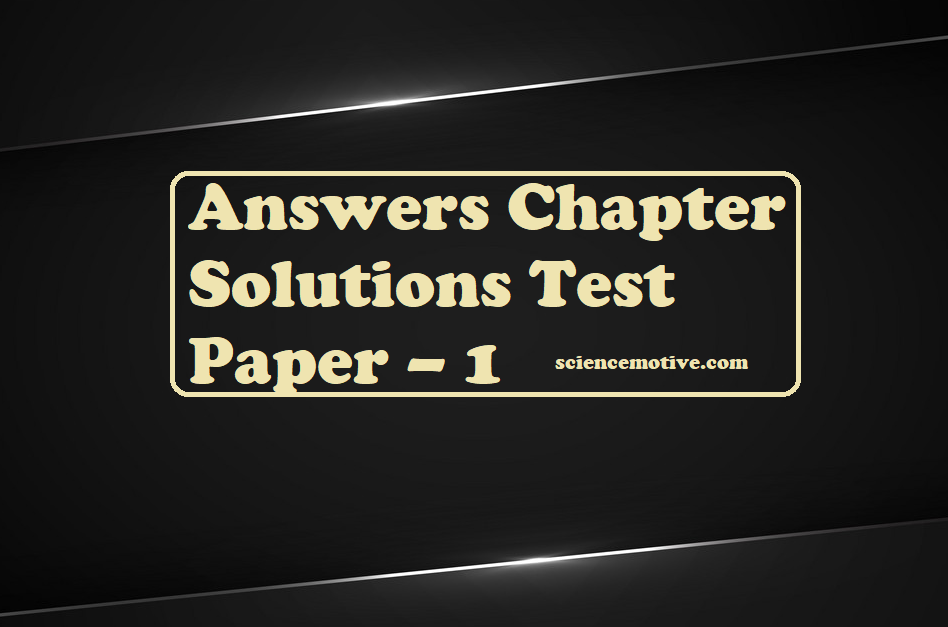# Answers Chapter Solutions Test Paper – 1

Ans 1. (C).
Explanation: Let, mass of solution = 100 g
Mass of nitric acid in the solution = 68 g
Density of the solution = 1.504 g mL–1
Volume of the solution = 100 g/1.504 g/ml
= 66.49 mL =  66.49/ 1000L = 0.066 L
Amount of nitric acid in solution
= 68g / Molar mass of nitric acid
= 68 g / 63g/mol
= 1.079 mol
Then, molarity of nitric acid solution
= 1.079 / 0.066 = 16.23 mol/L

Ans 2. (B).
Explanation: Level of contamination = 15 ppm
= 15 parts in 106 parts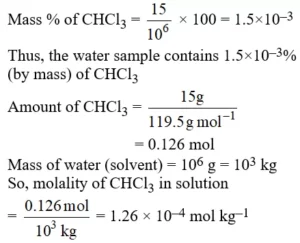Ans 3. (B).
Explanation: This Shows a negative deviation from Raoult’s law. If the attraction between different molecules, for example between HCl and H2O molecules, is stronger, the escaping tendency from the solution to the vapour phase will be smaller. As a result, the partial vapour pressure will be smaller than predicted by Raoult’s law and the system exhibits a negative deviation.

Ans 4. (A)
Explanation: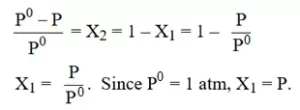Ans 5. (C).
Explanation: x × 92.6 = (1 – x) 140.9 = 140.9 – 140.9 x
92.6 x = 140.9 – 140.9 x
140.9 x + 92.6 x = 140.9
232.6 x = 140.9
x = 140.9/232.6
x = 0.605 = mole fraction of A

Ans 6. (C).
Explanation: Molality: It is defined as the number of gram moles present in one kg of solvent. This involves mass rather than volume. This is independent of variation in temperature.

Ans 7. (B).
Explanation: Π = CRT where C is the molarity of the solution. Hence, osmotic pressure is only associated with the molarity of the solution.

Ans 8. (A).
Explanation: p0 = 17.535 mm Hg,  ps = ?
Mass of glucose = 25g,
Molar mass of glucose = 180 g/mol,
Mass of water = 450 g
The molar mass of water = 18 g/mol
Mole fraction of glucose in solution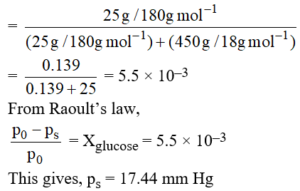Ans 9. (C).
Explanation: Number of moles of urea = 1 mole
Number of moles of solvent is 1000 gms of EtOH = 21.7
Total number of moles = 22.7
Mole fraction = 1/22.7 = 0.044.

Ana 10. (A).
Explanation: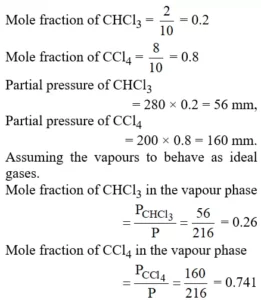Ans 11. (A).
Explanation: Cane sugar solution:
Let the Mass of the solution = 100 g
So, the mass of cane sugar = 5g,
So, mass of water = 95 g = 0.095 kg
Molar mass cane sugar = 342 g mol–1
Thus, the molality of the solution,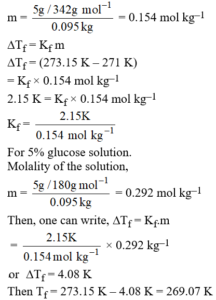Ans 12. (A).
Explanation: Π α No. of partial/ion
BaCl2 = 3,  NaCl = 2,   glucose = 1
So, order of Π = BaCl2 > NaCl > glucose

Ans 13. (C).  The no. of species obtained after the complete dissociation of Al2(SO4)3 is the same as the no. of species obtained after the complete dissociation of K4[Fe(CN)6].

Ans 14. (A)
Explanation: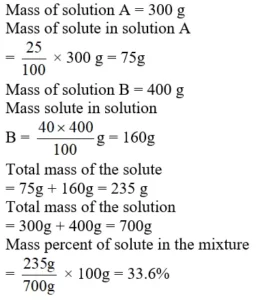Therefore, mass percent of solvent in the mixture = (100 – 33.6)% = 66.4%

Ans 15. (A).
Explanation: Heat associated with melting of ice
= 6.01 KJ mol–1
1 of mol. of ice given = 25/ 18 = 1.389
Hence total heat is associated with the melting of ice for a given quantity of ice
= 1.389 × 6.01 = 8.347 KJ
Heat lost when 100 gm of liquid water at 22ºC is cooled to 0ºC
= 100 × 4.18 × (–22) = – 9196 J
= – 9.196 KJ
Thus 9.196 KJ is greater than that required to melt all ice. Hence then is “all liquid” above 0ºC.
Let final temperature = TºC
The heat associated with melting of 25gm ice at 0ºC = 8.847 KJ = 8347 J
Heat associated to convert liquid at 0ºC to liquid at TºC = 25 × 4.18 × (T – 0) = 104.5 T.
Heat released when 100 gm of water is cooled from 22ºC to TºC
= 100 × 4.18 × (22 – T) = 9196 – 418 T
Thus heat gained = heat lost
8347 + 104.5T = 9196 – 418 T
= 522.5 T = 849 ; T = 1.625ºC.

Ans 16. (B).
Explanation: Mole fraction of ether in the liquid mixture
= (1 – 0.457) = 0.543
Partial pressure of ether = 0.543 × 443.5 = 240.78 mm

Ans 17. (A).
Explanation: A dilute solution may be defined as one in which the solvent obeys Raoult’s law.

Ans 18. (D).
Explanation: The solution with the lowest freezing point corresponds to the one with the most particles in the solution.
Lithium sulphate puts three ions in solution per lithium sulphate formula unit.

Ans 19. (C).
Π = CRT
2.47 = C × 0.082 × 303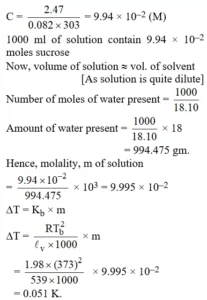Ans 20. (A)
Explanation: 98% by weight H2​SO4​ = 98 g H2​SO4​​/100 g solution
Volume of 100 g solution = mass/density​ = 100/1.8 ​ = 55.55 mL
For, 0.1 M, we need 9.8 g H2​SO4
55.55 mL acid = 98 g
5.55 ml acid = 9.8 g = 0.1 Molar acid

Ans 21. (B)
Explanation: An aqueous solution is 1.00 molal in KI. So, the addition of water will cause the vapour pressure to increase. This is according to Raoult’s Law.
In the other three options, electrolytes undergo ionization, which leads to a lowering of vapour pressure.

Ans 22. (a), (c)

Ans 23. (A)

Ans 24. (A)

To get Questions Click the link below:

Chapter Solutions Test Paper – 1